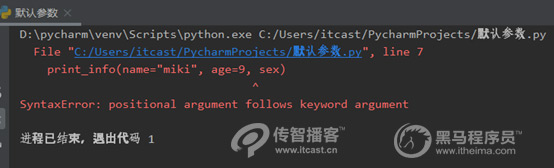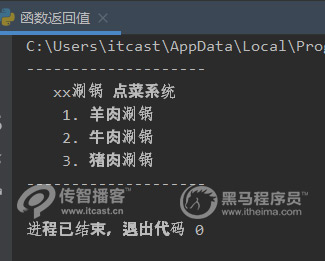# python函数是什么，怎么用？【python培训】

1、什么是函数？
2、如何定义函数？
3、如何调用函数？
4、函数参数传递
5、函数默认参数
6、不定长参数
7、函数的返回值
8、函数的四种类型1、什么是函数？

Python提供了很多内建函数，如print()。除此之外,我们还可以自己创建函数,也就是自定义函数。接下来，看一段代码：
print("*")
print("**")
print("****")

2、如何定义函数？

def 函数名（参数列表）：

“函数_文档字符串”

函数体

return 表达式

(1)函数代码块以def开头，后面紧跟的是函数名和圆括号()。
(2)函数名的命名规则跟变量的命名规则是一样的，即只能是字母、数字和下划线的任何组合，但是不能以数字开头，并且不能跟关键字重名。
(3)函数的参数必须放在圆括号中。
(4)函数的第1行语句可以选择性地使用文档字符串来存放函数说明。
(5)函数内容以冒号起始，并且缩进。
(6) return表达式结束函数，选择性地返回一个值给调用方。不带表达式的retu相当于返回None。

3、如何调用函数？

# 定义一个函数，能够完成打印信息功能
def print_info():
print("-"*20)
print("人生苦短，我用python！")
print("-"*20)
# 调用函数
print_info()

4、函数参数传递

c = 11 + 22
print(c)

c = a + b
print(c)

c = a + b
print(c)
# 调用带有参数的函数时，需要在小括号中传递数据。

50

5、函数默认参数

def print_info(name, age=35):
# 打印任何传入的字符串
print("Name：", name)
print("age：", age)
# 调用print_info(name="miki")
print_info(name="miki")
print_info(name="miki", age=9)

Name： miki
age： 35
Name： miki
age： 96、不定长参数

def 函数名([forml_args,] *args, **kwargs):
"函数_文档字符串"
函数体
return 表达式

（1）如果传入的参数没有指定名称，那么*args会以元组的形式存放这些多余的参数
（2）如果传入的参数指定了名称，如m=1，那么* kwargs会以字典的形式存放这些被命名的参数，如{m:1}。

def test(a, b, *args):
print(a)
print(b)
print(args)
test(11, 12)

11
12
()

def test(a, b, *args):
print(a)
print(b)
print(args)
test(11, 12, 13, 14, 15, 16, 17, 18)

11
12
(13, 14, 15, 16, 17, 18)

def test(a, b, *args, **kwargs):
print(a)
print(b)
print(args)
print(kwargs)
test(11, 12, 13, 14, 15, 16, 17, 18)

11
12
(13, 14, 15, 16, 17, 18)
{}

def test(a, b, *args, **kwargs):
print(a)
print(b)
print(args)
print(kwargs)
test(11, 12, 13, 14, 15, 16, 17, 18, m=22, n=33)

11
12
(13, 14, 15, 16, 17, 18)
{'m': 22, 'n': 33}

7、函数的返回值

c = a + b
return c

return a + b

8、函数的四种类型

（1）函数无参数，无返回值。
（2）函数无参数，有返回值。
（3）函数有参数，无返回值。
（4）函数有采参数，有返回值。

1）无参数、无返回值的参数

print("-"*20)
print("   xx涮锅 点菜系统")
print("    1. 羊肉涮锅")
print("    2. 牛肉涮锅")
print("    3. 猪肉涮锅")
print("-" * 20)2）无参数、有返回值的函数

# 获取温度
def get_temperature():
# 这里是温度获取的一些处理过程
# 为了简单起见，先模拟返回一个数据
return 24
temperture = get_temperature()
print('当前的温度为：', temperture)

3）有参数、无返回值的函数

def test(number_one, number_two):
result = number_one + number_two
print('计算结果为： %d'%result)

4）有参数、有返回值得函数

# 计算1~number的累积和
def calculate(nuber):
result = 0
i = 1
while i <= nuber:
result = result +i;
i +=1
return result
result = calculate(100)
print('1~100的累积和为：', result)

1~100的累积和为： 5050

## 相关阅读